# RD Sharma Solutions for Class 11 Chapter 10 - Sine and Cosine Formulae and their Applications Exercise 10.1

Exercise 10.1 of Chapter 10, we shall discuss problems based on the law of sines or sine rule. Highly experienced subject experts at BYJU’S having vast knowledge of concepts develop the solutions, which match the understanding ability of the students. Students can use RD Sharma Class 11 Solutions for Maths as the best study material which consists of solutions explained in the most simple language which any student can understand. The solutions of this exercise are made available in the RD Sharma Class 11 Maths pdf, which can be downloaded easily from the below given links.

## Download the Pdf of RD Sharma Solutions for Class 11 Maths Exercise 10.1 Chapter 10 – Sine and Cosine Formulae and their Applications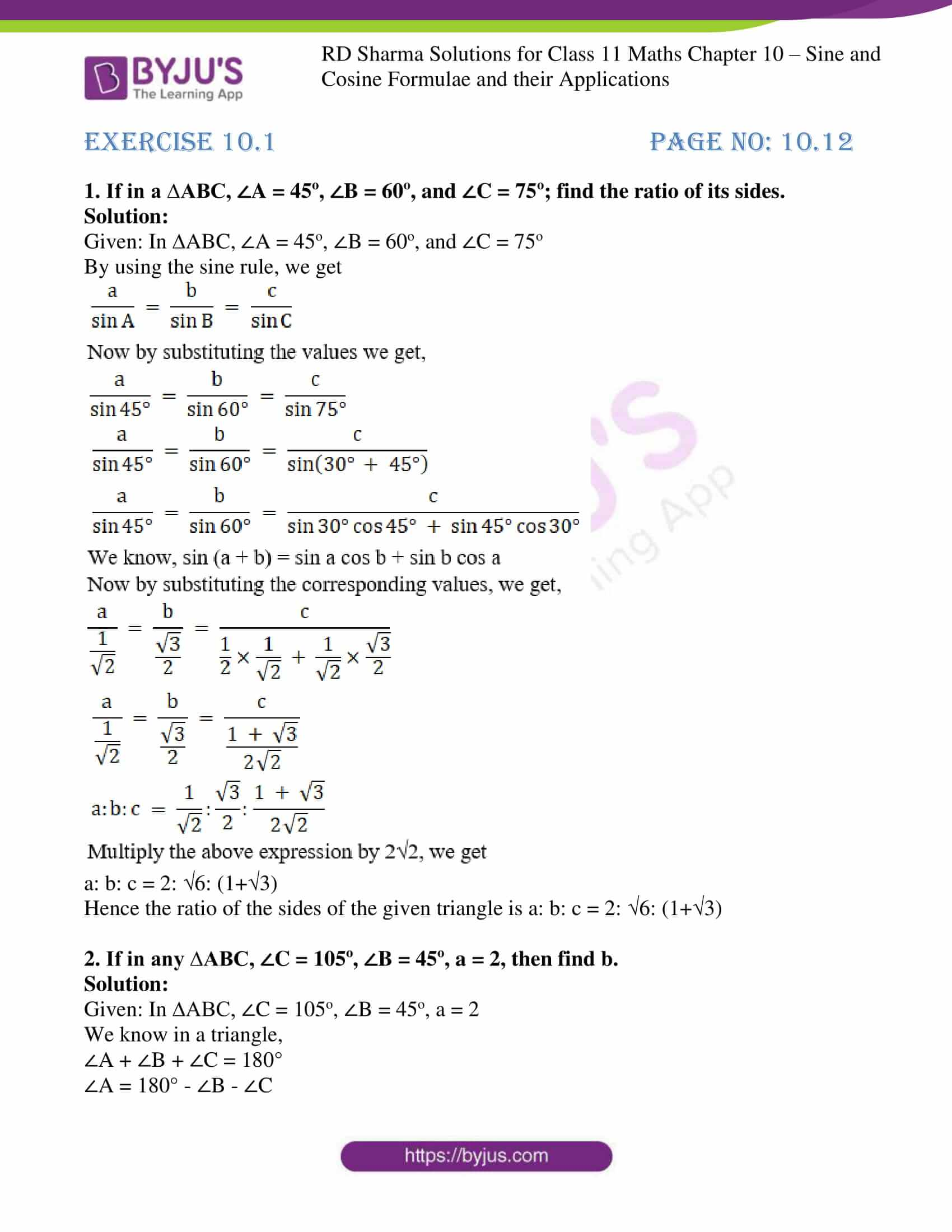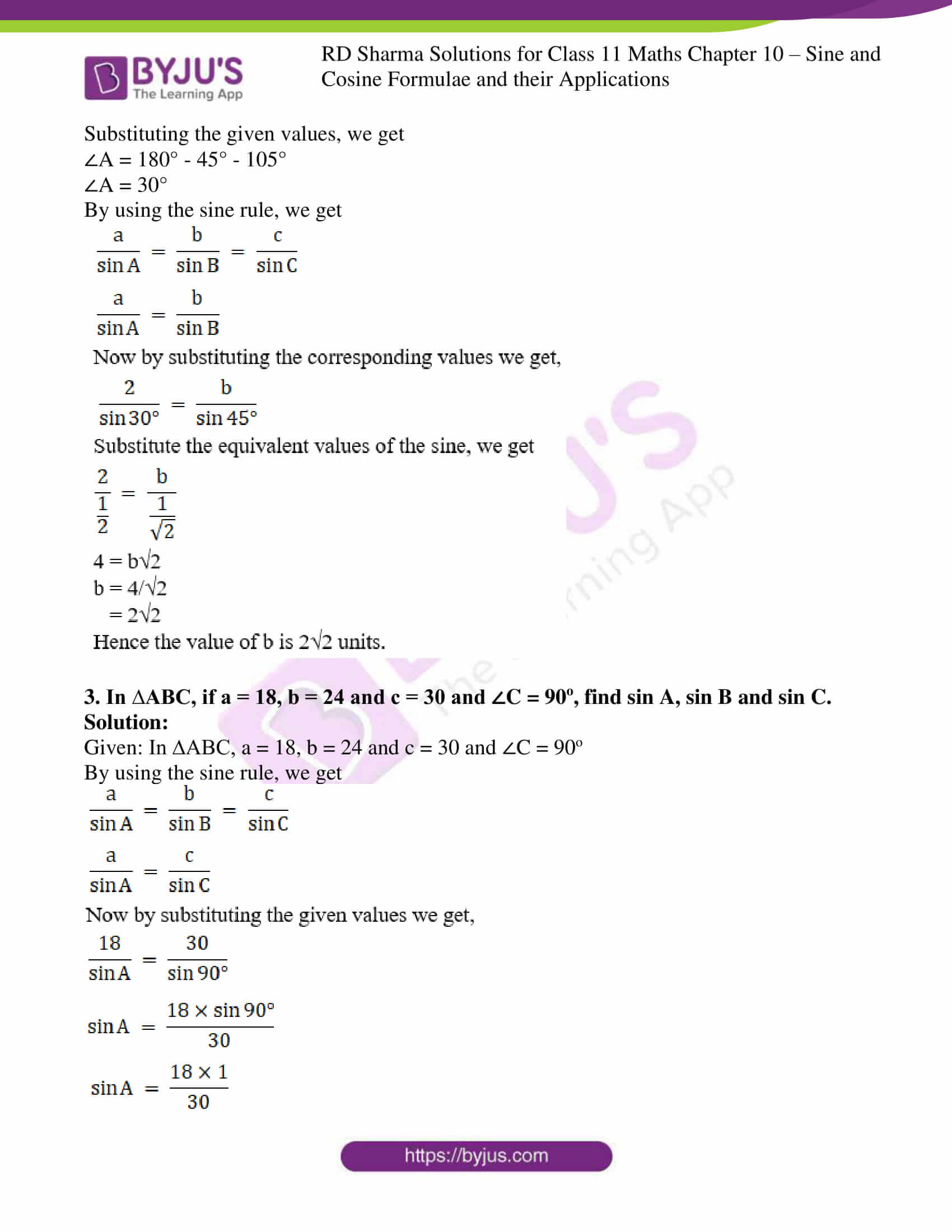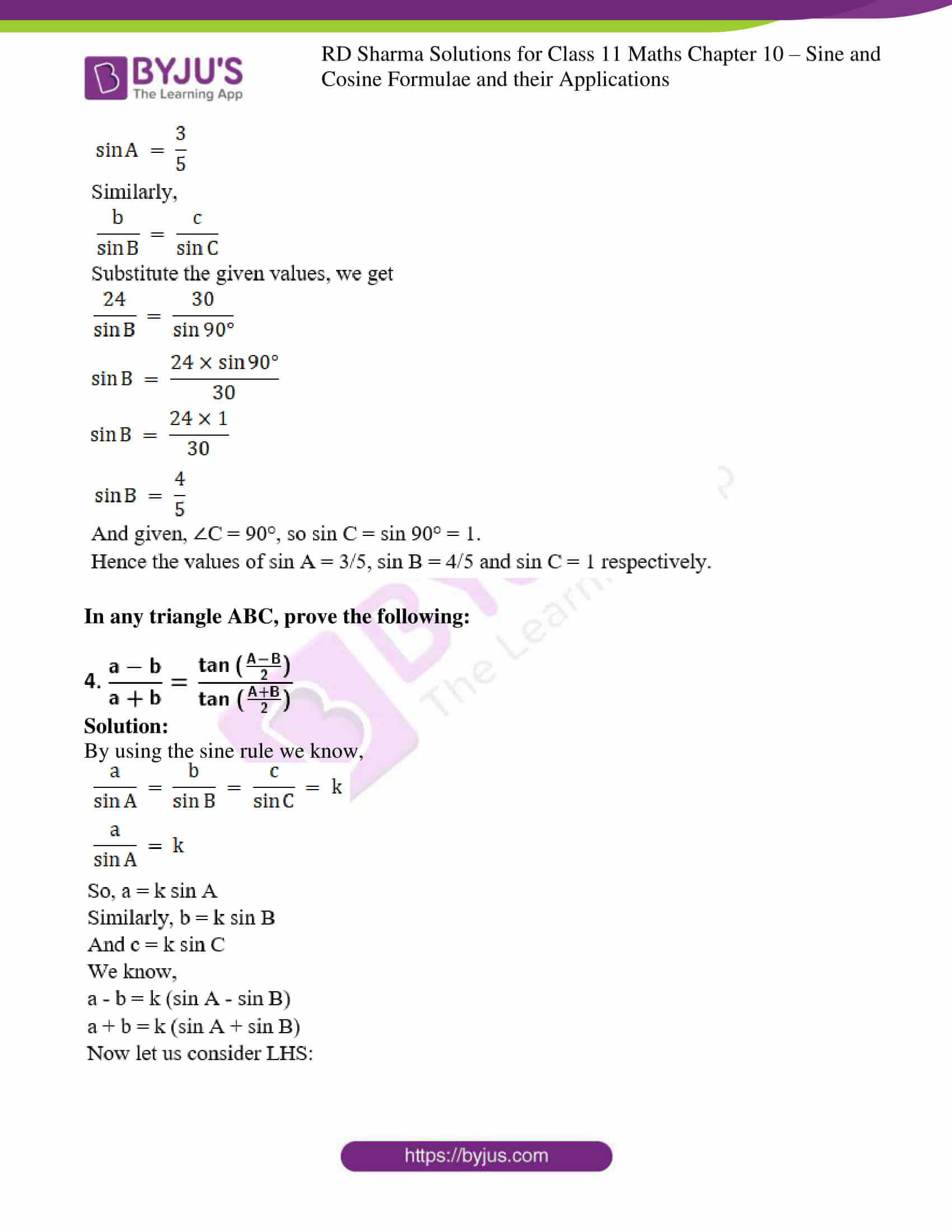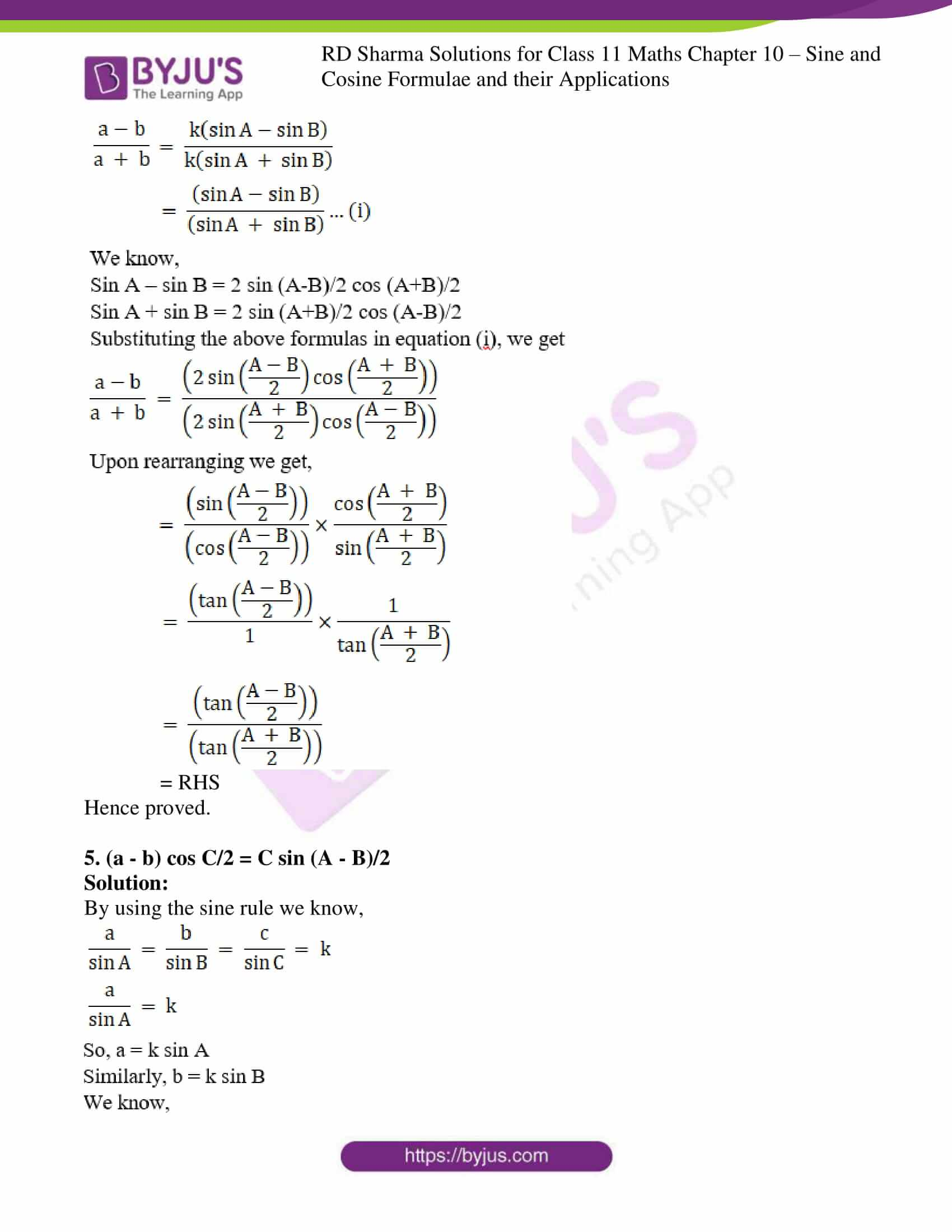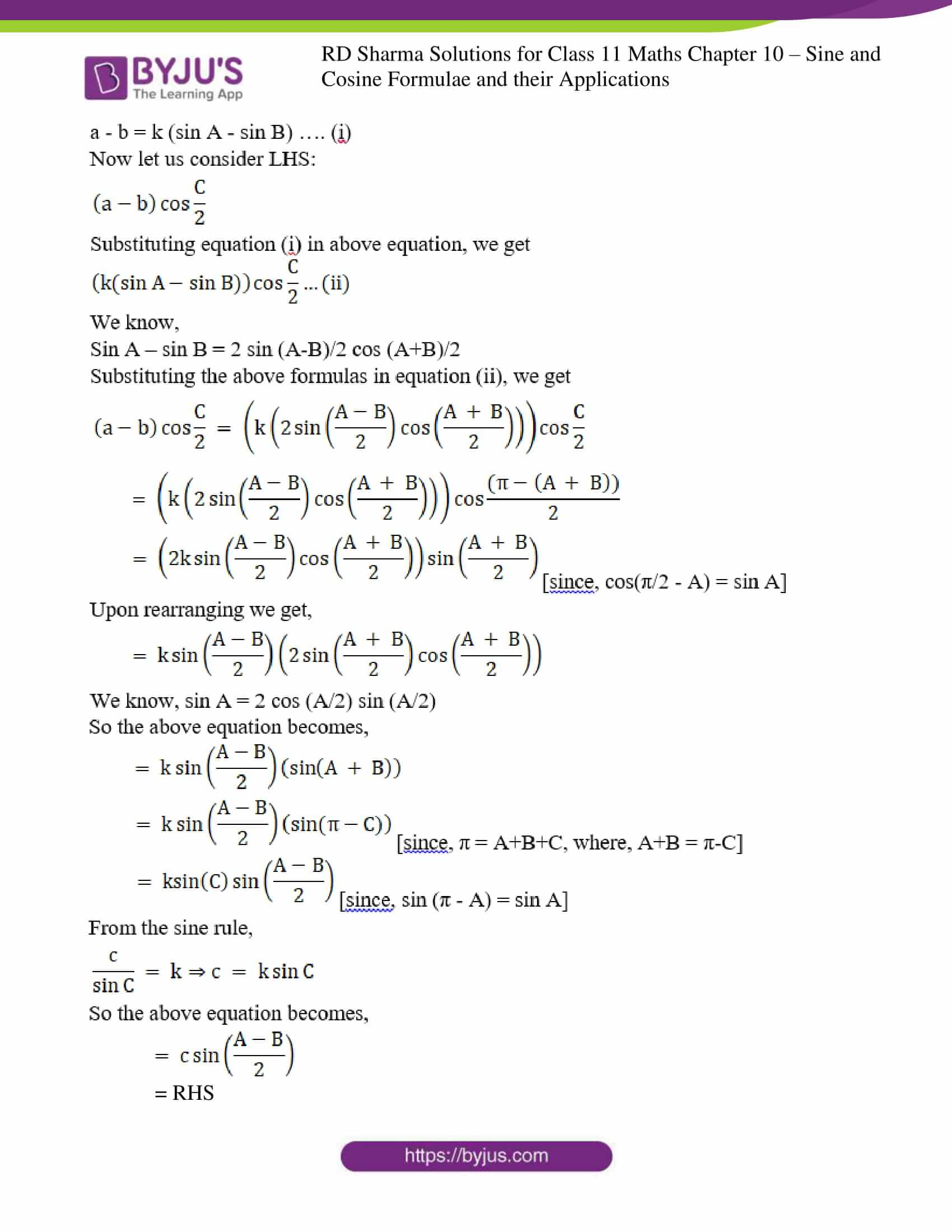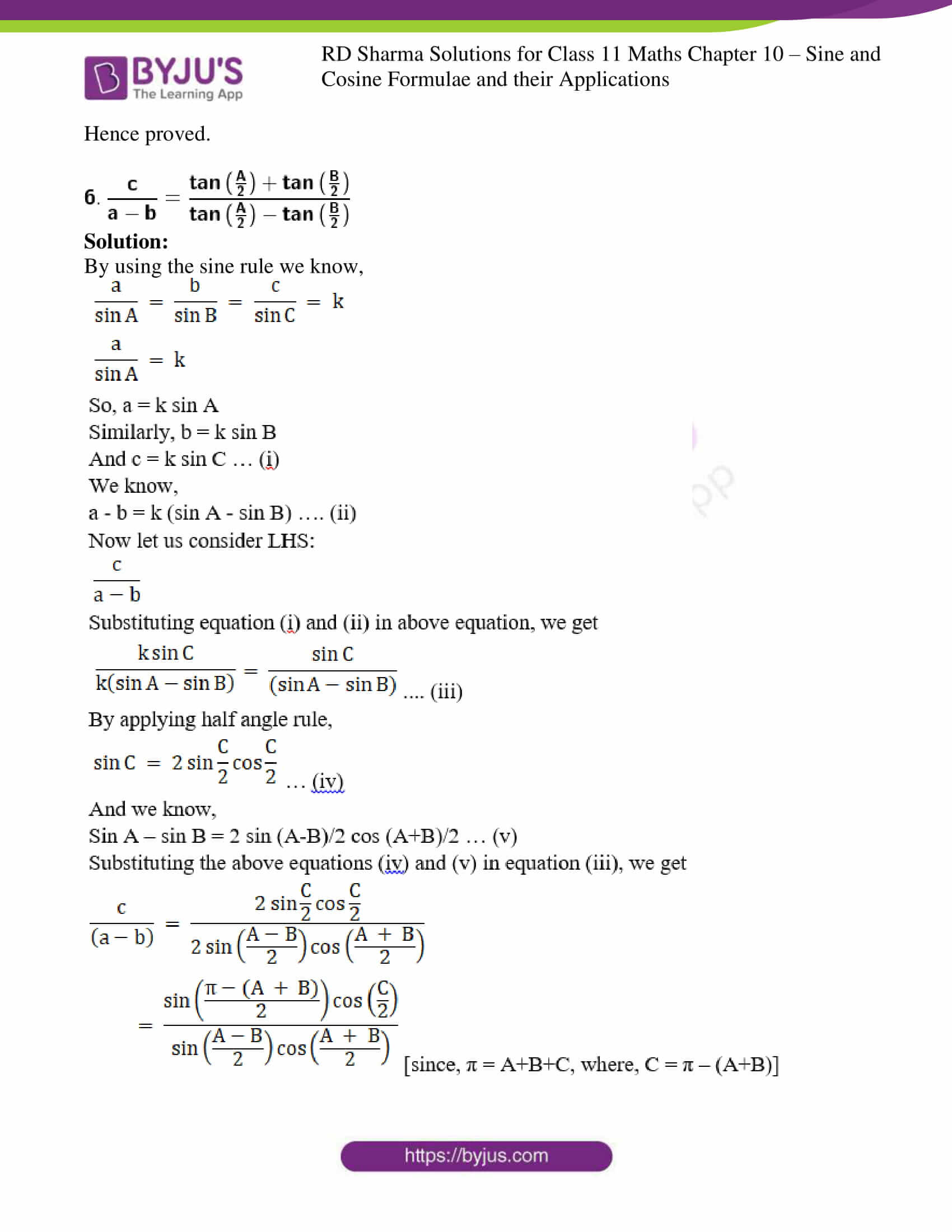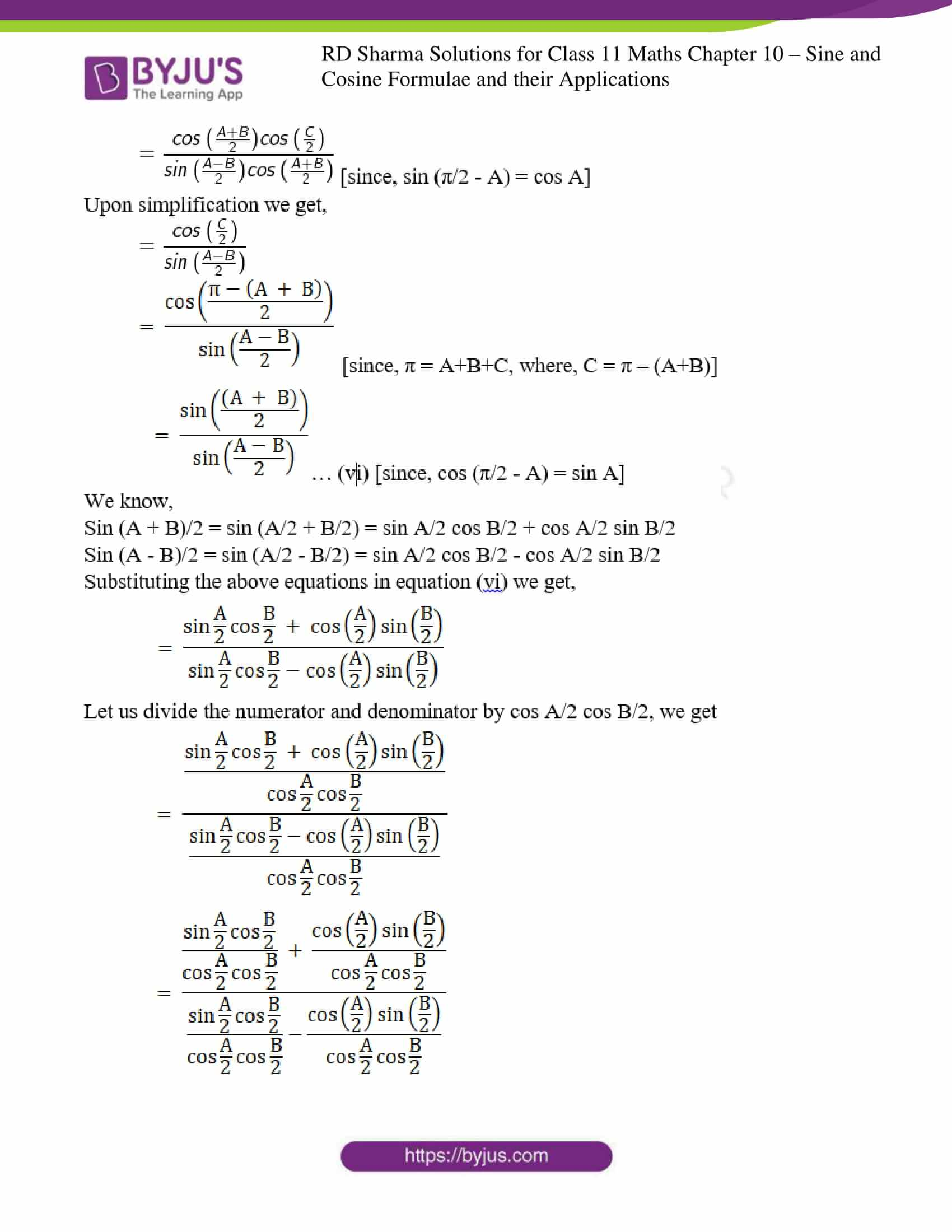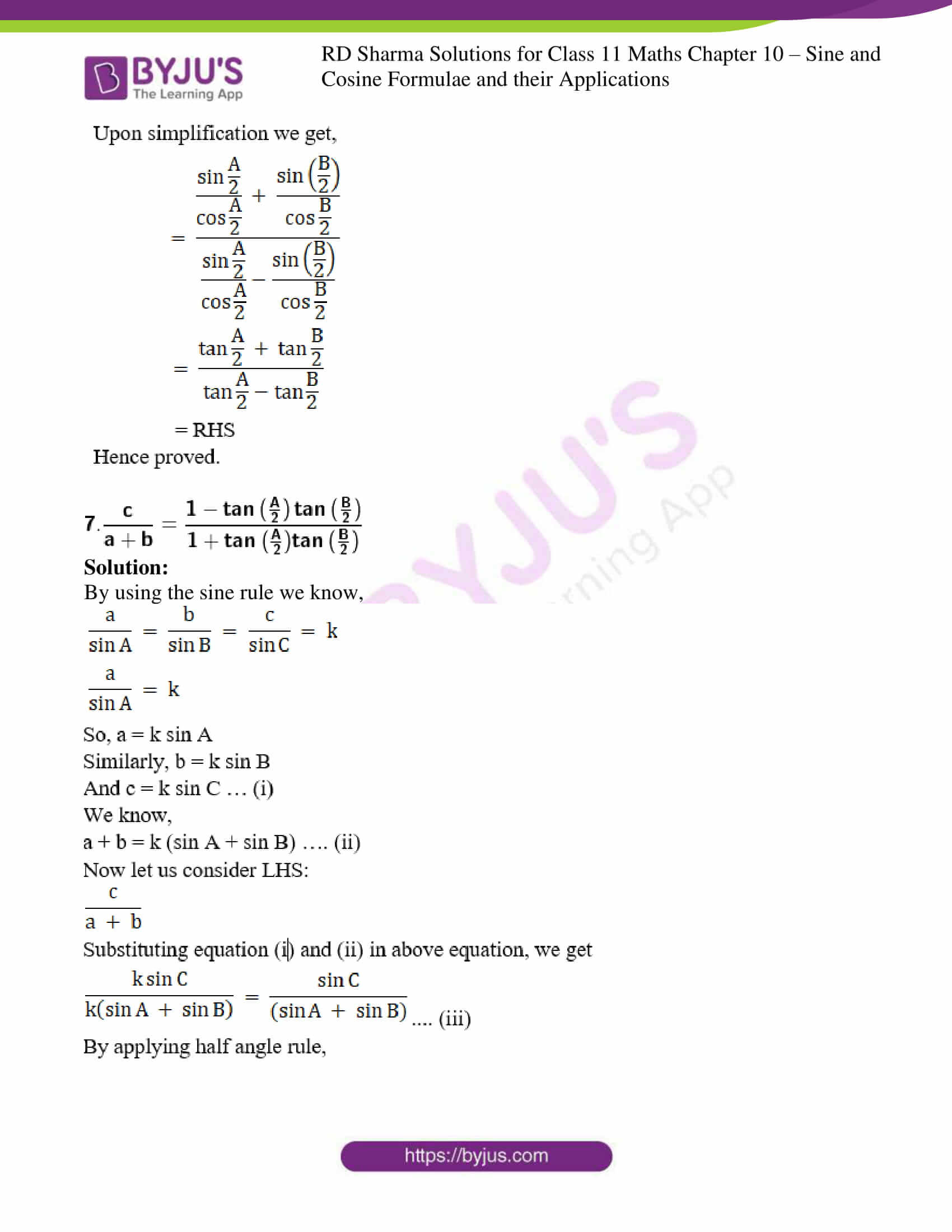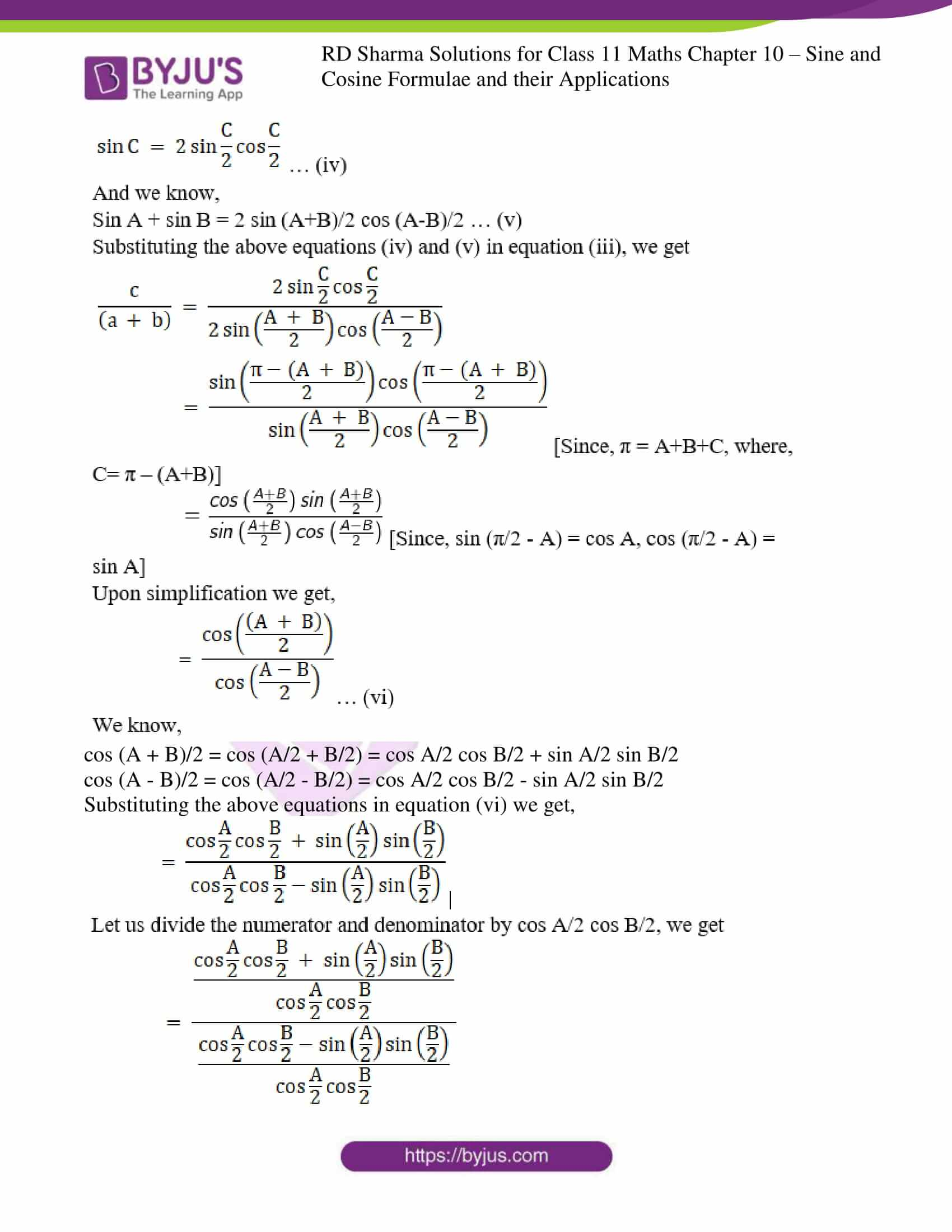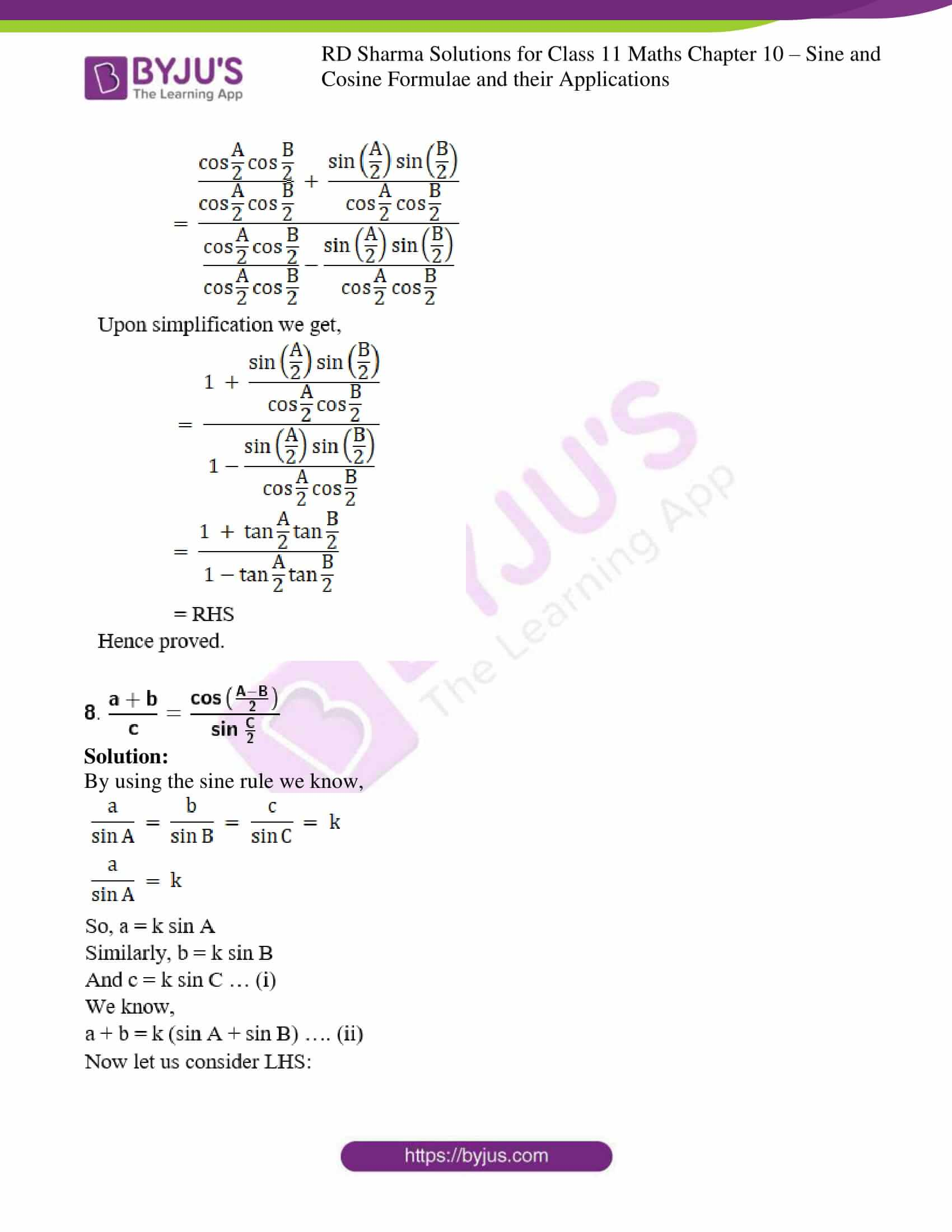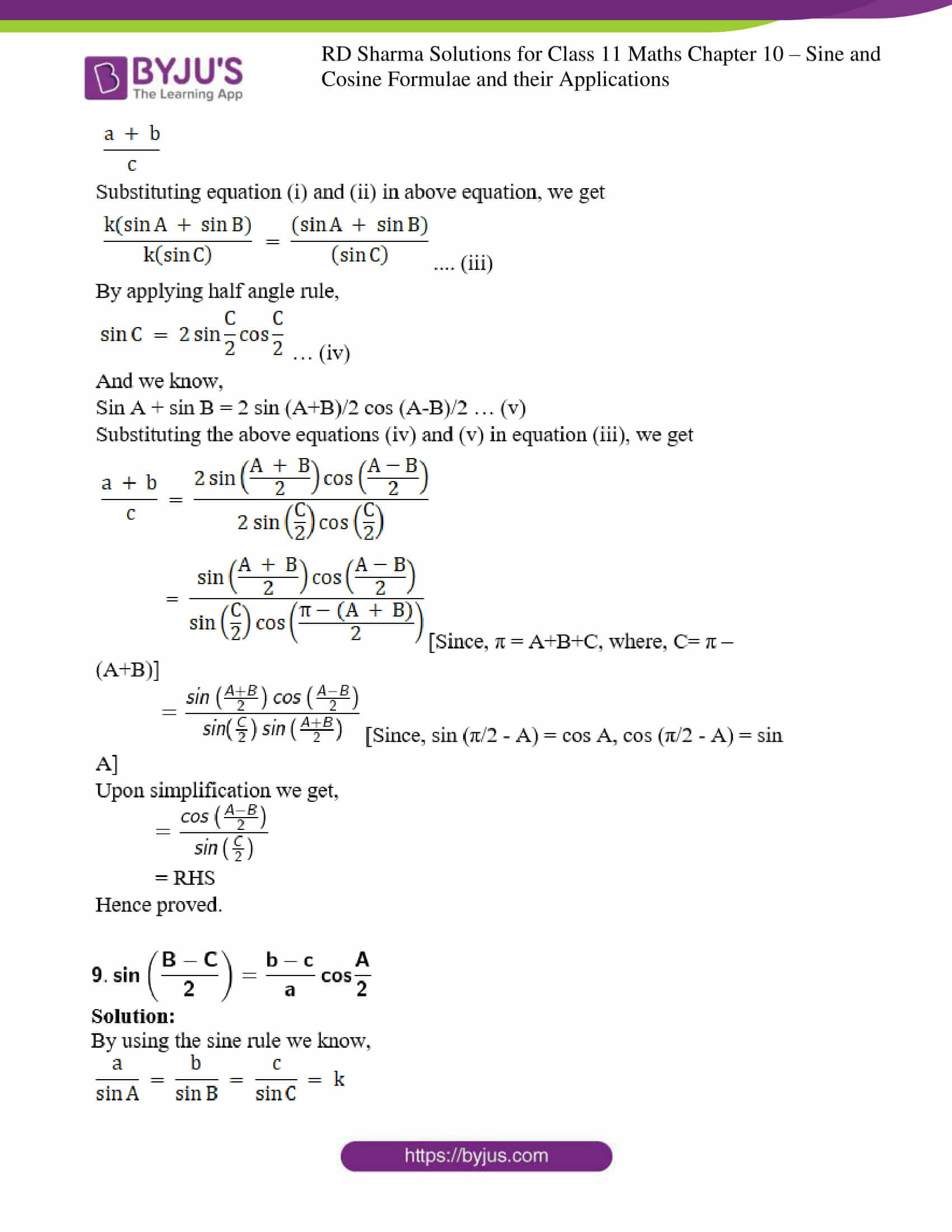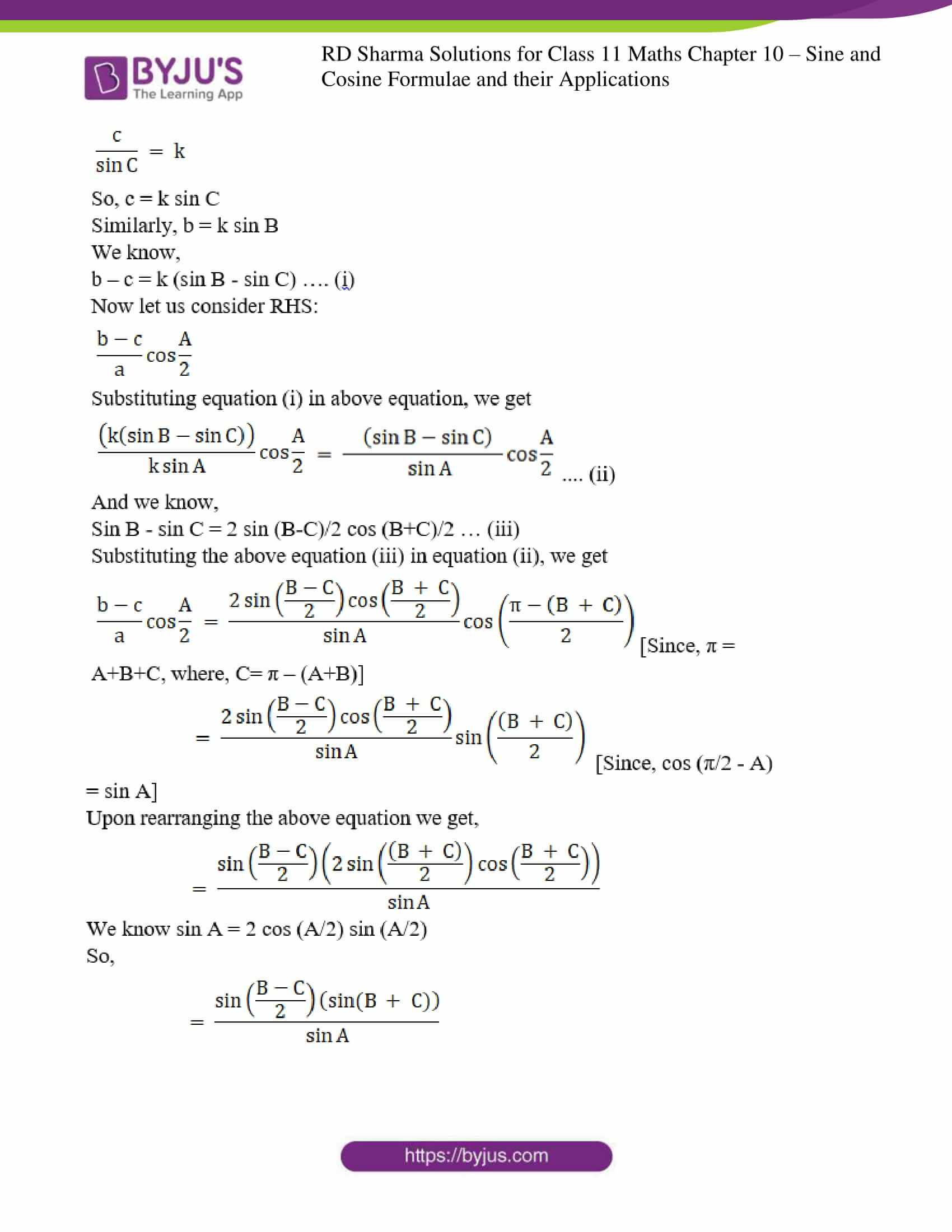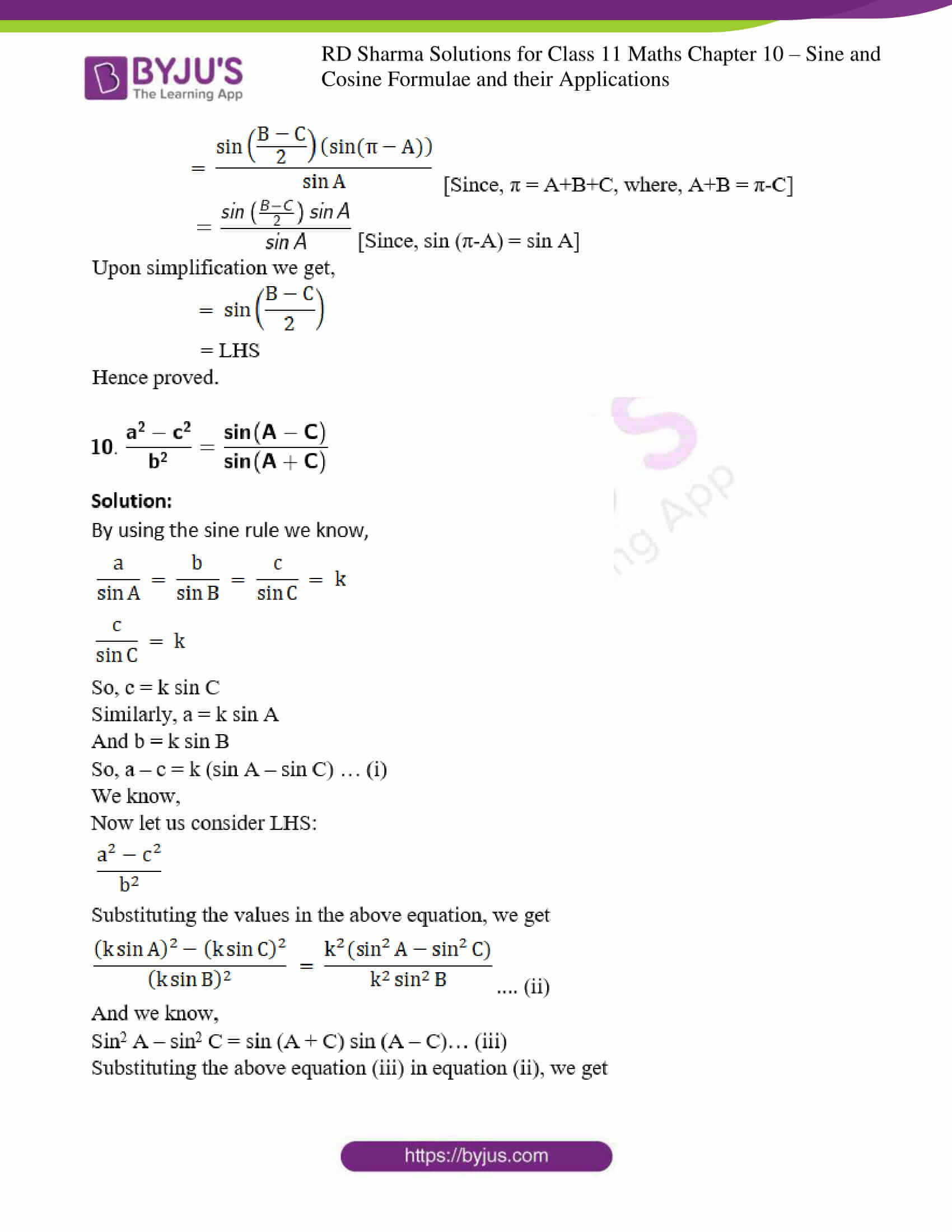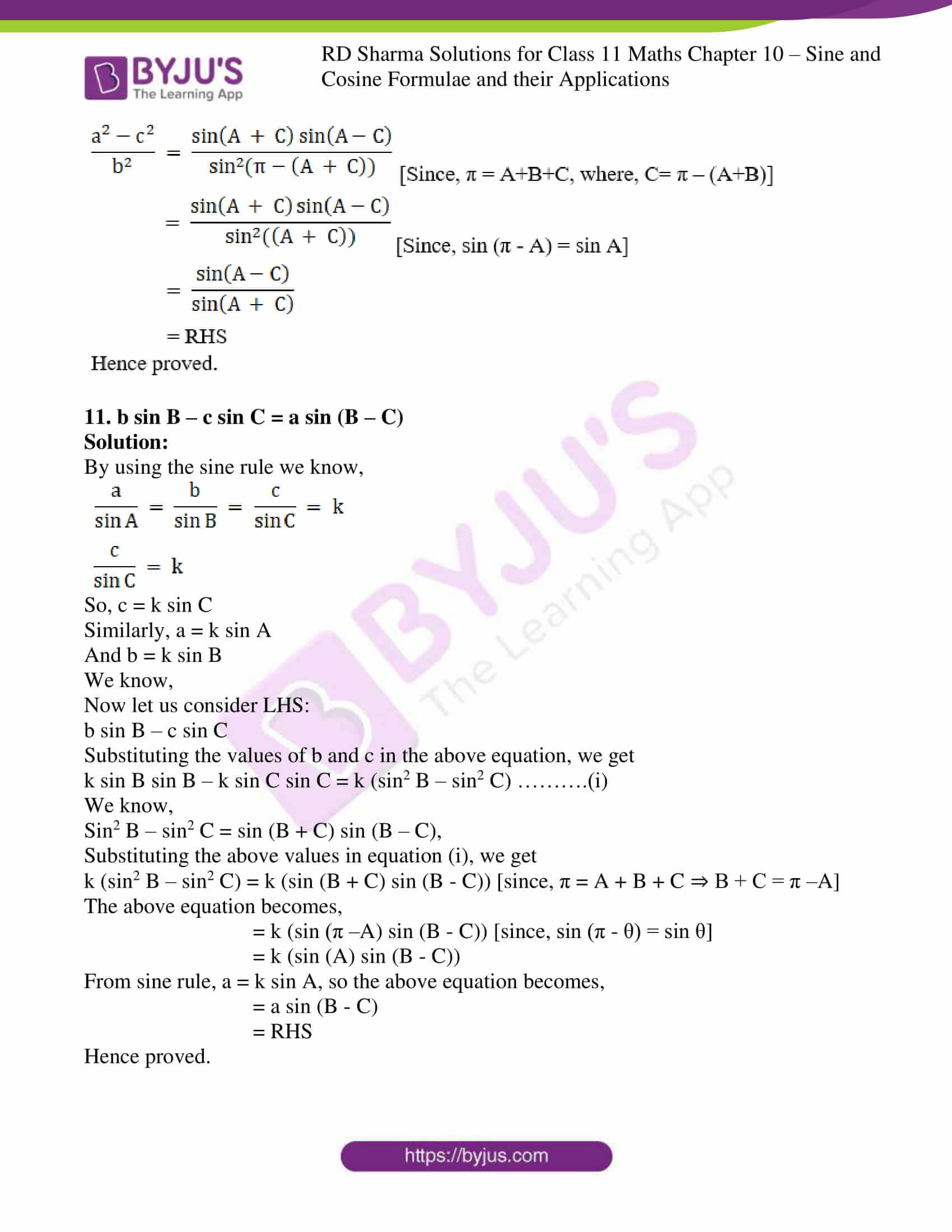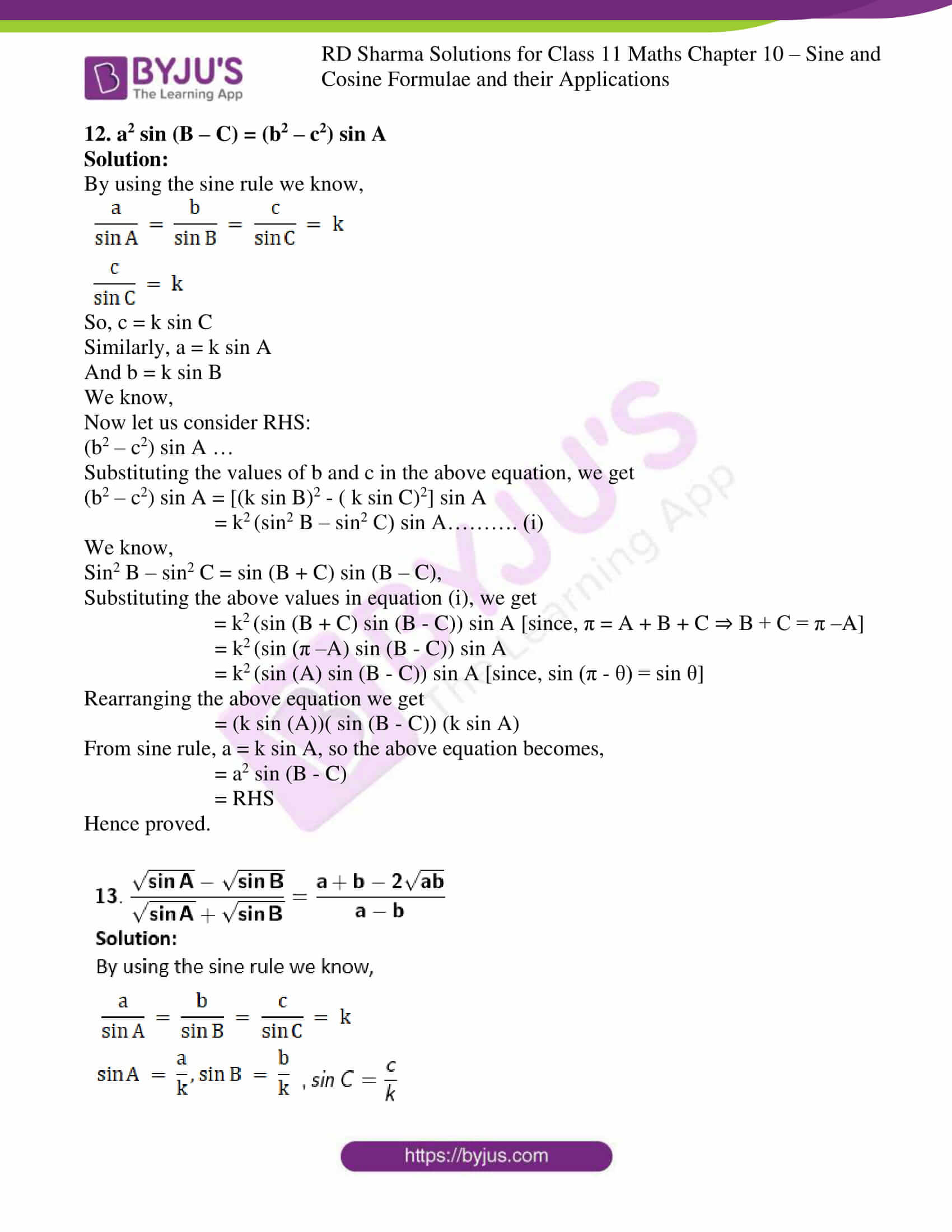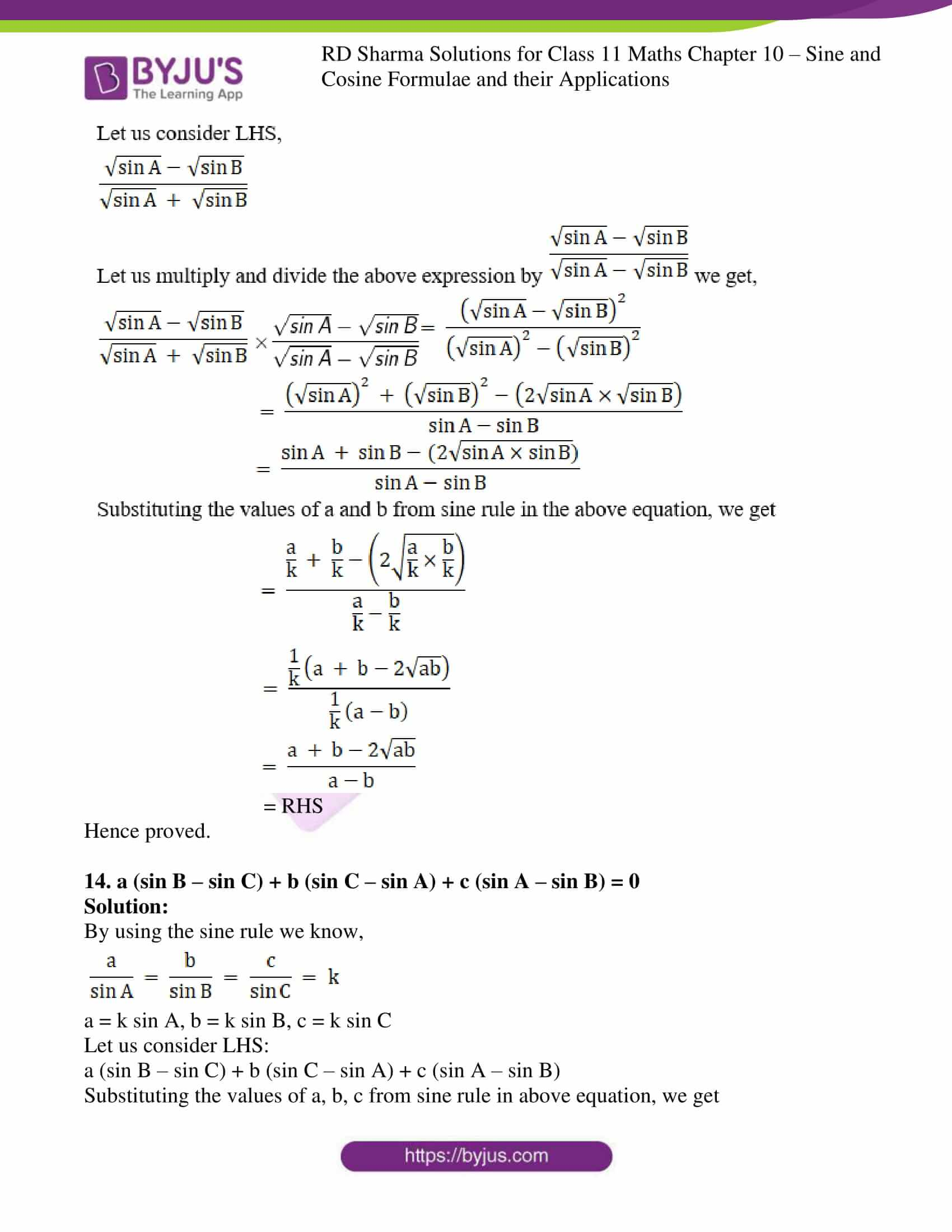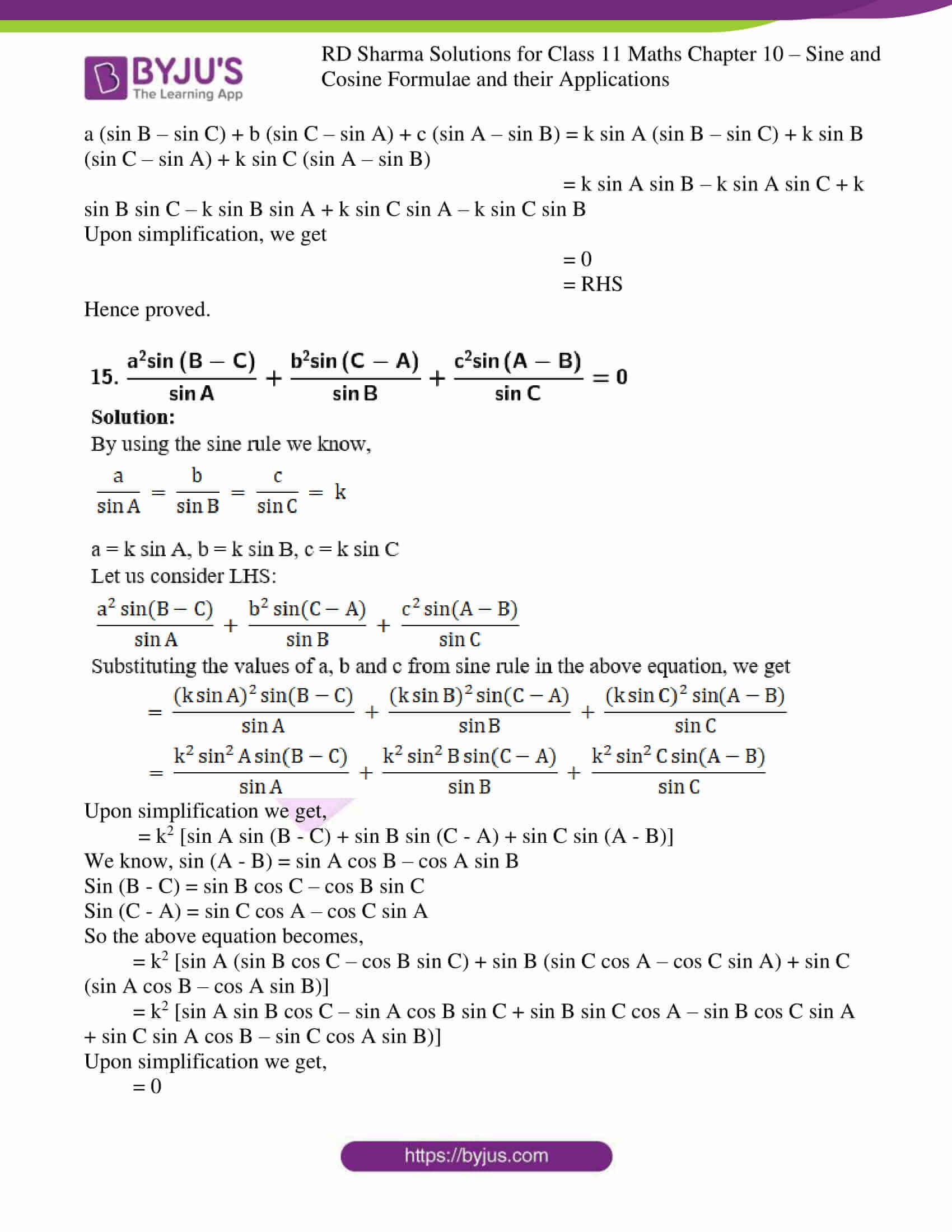### Access answers to RD Sharma Solutions for Class 11 Maths Exercise 10.1 Chapter 10 – Sine and Cosine Formulae and their Applications

1. If in a ∆ABC, ∠A = 45o, ∠B = 60o, and ∠C = 75o; find the ratio of its sides.

Solution:

Given: In ABC, ∠A = 45o, ∠B = 60o, and ∠C = 75o

By using the sine rule, we get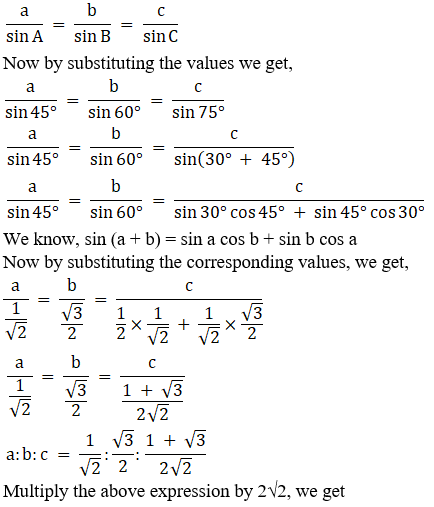a: b: c = 2: √6: (1+√3)

Hence the ratio of the sides of the given triangle is a: b: c = 2: √6: (1+√3)

2. If in any ∆ABC, ∠C = 105o, ∠B = 45o, a = 2, then find b.

Solution:

Given: In ∆ABC, ∠C = 105o, ∠B = 45o, a = 2

We know in a triangle,

∠A + ∠B + ∠C = 180°

∠A = 180° – ∠B – ∠C

Substituting the given values, we get

∠A = 180° – 45° – 105°

∠A = 30°

By using the sine rule, we get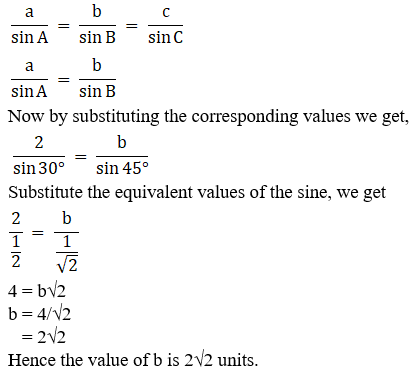3. In ∆ABC, if a = 18, b = 24 and c = 30 and ∠C = 90o, find sin A, sin B and sin C.

Solution:

Given: In ∆ABC, a = 18, b = 24 and c = 30 and ∠C = 90o

By using the sine rule, we get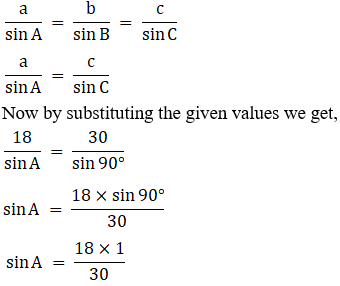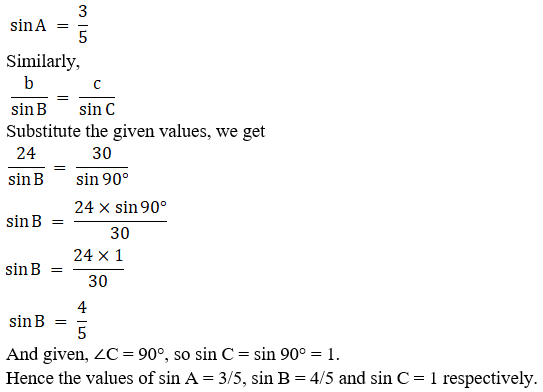In any triangle ABC, prove the following: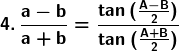Solution:

By using the sine rule we know,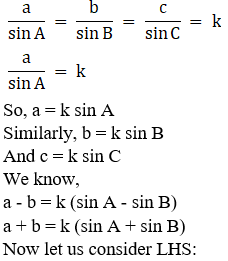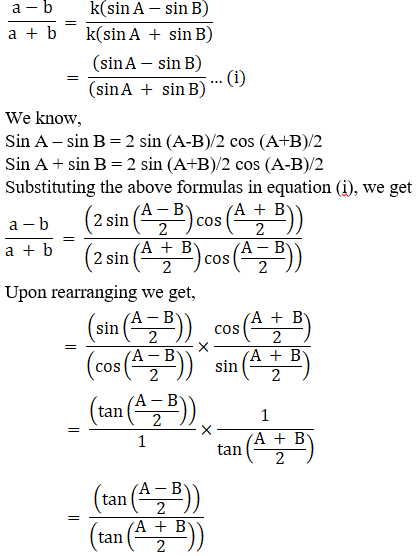= RHS

Hence proved.

5. (a – b) cos C/2 = C sin (A – B)/2

Solution:

By using the sine rule we know,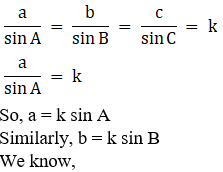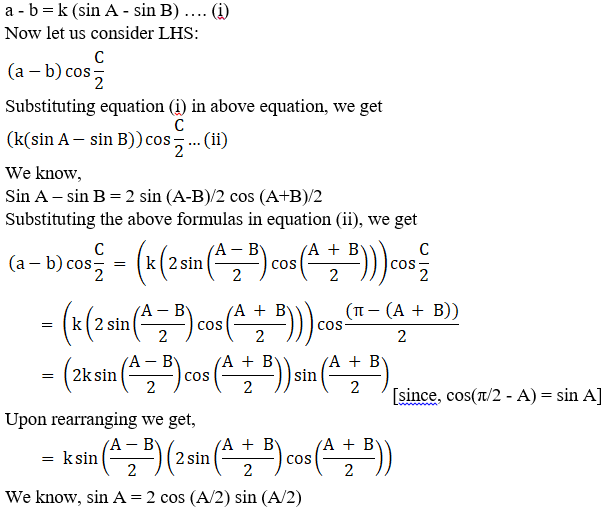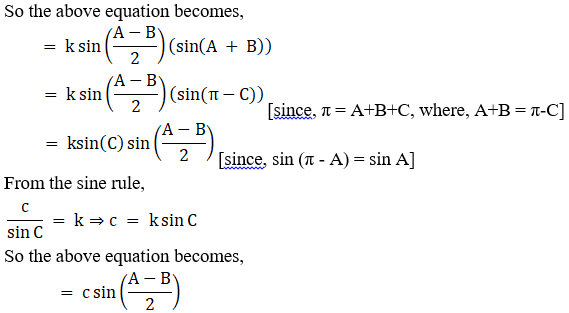= RHS

Hence proved.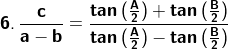Solution:

By using the sine rule we know,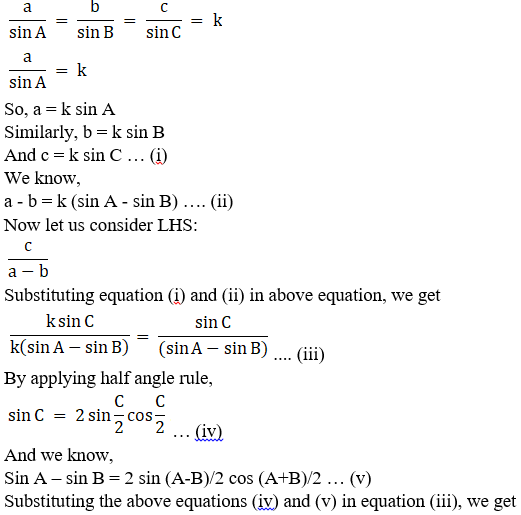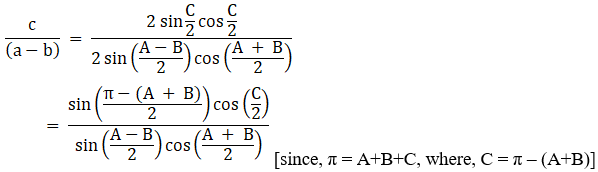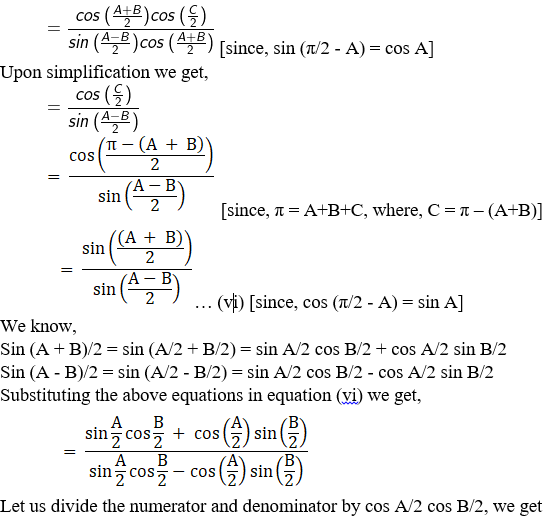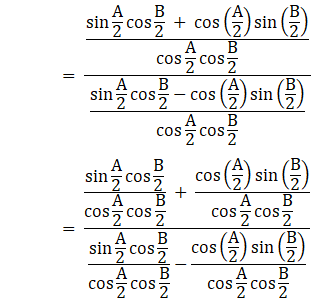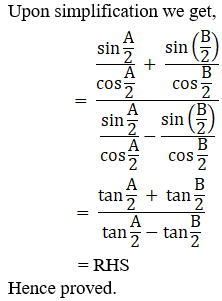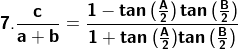Solution:

By using the sine rule we know,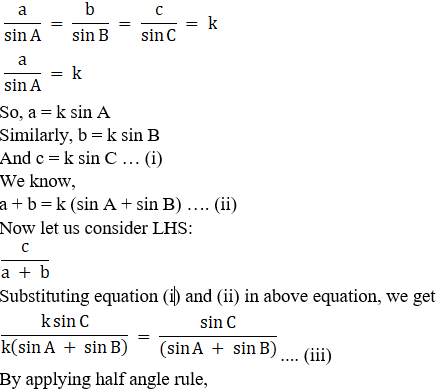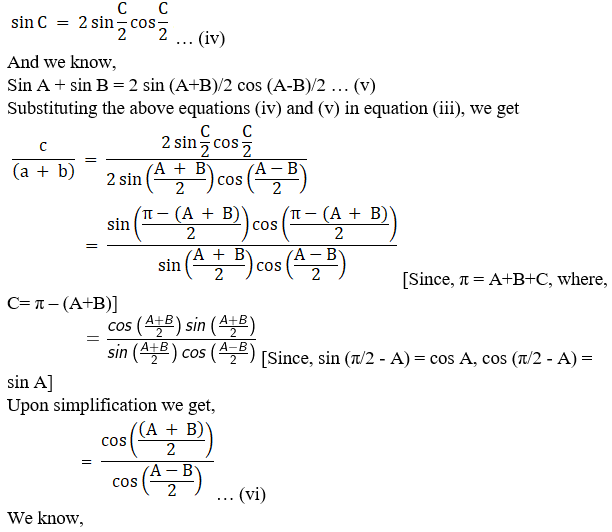cos (A + B)/2 = cos (A/2 + B/2) = cos A/2 cos B/2 + sin A/2 sin B/2

cos (A – B)/2 = cos (A/2 – B/2) = cos A/2 cos B/2 – sin A/2 sin B/2

Substituting the above equations in equation (vi) we get,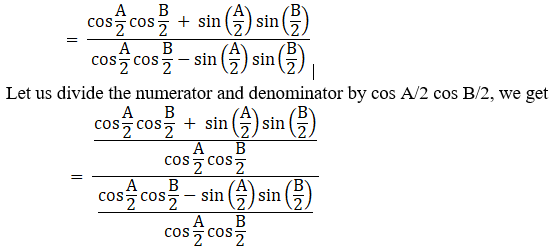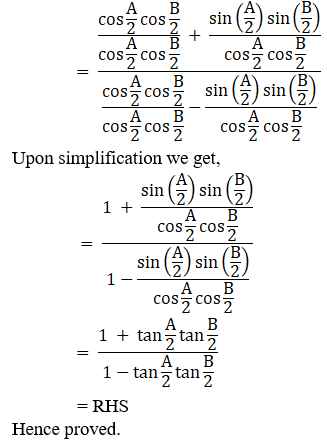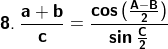Solution:

By using the sine rule we know,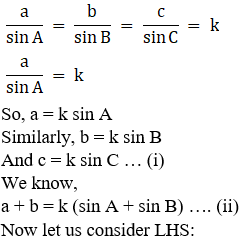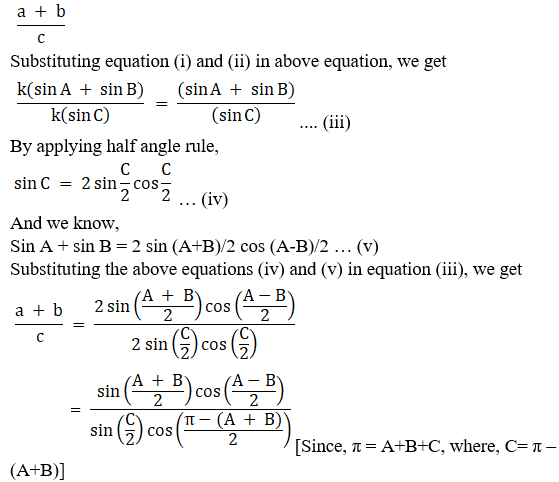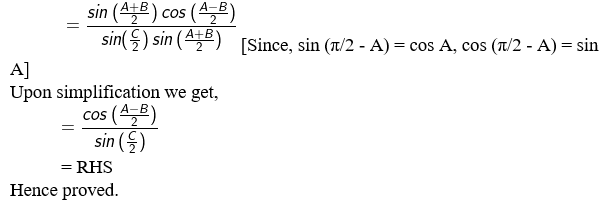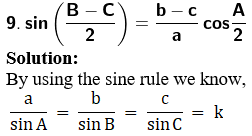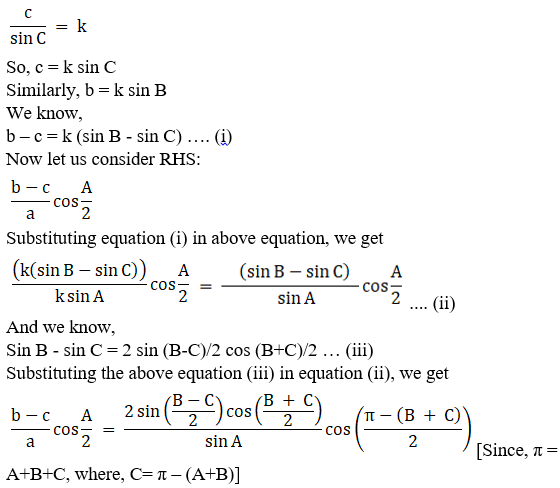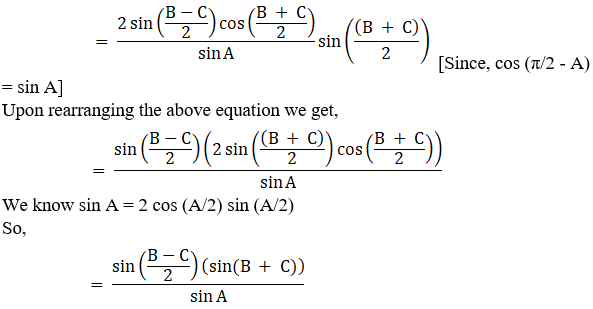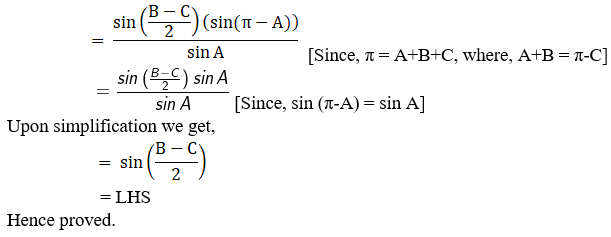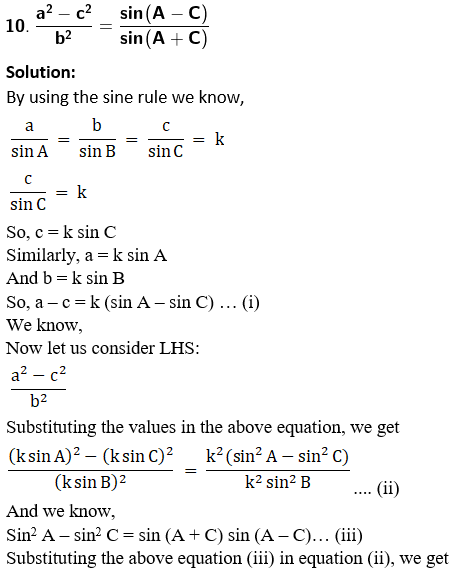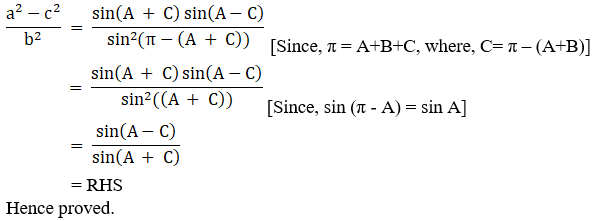11. b sin B – c sin C = a sin (B – C)

Solution:

By using the sine rule we know,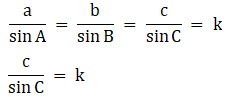So, c = k sin C

Similarly, a = k sin A

And b = k sin B

We know,

Now let us consider LHS:

b sin B – c sin C

Substituting the values of b and c in the above equation, we get

k sin B sin B – k sin C sin C = k (sin2 B – sin2 C) ……….(i)

We know,

Sin2 B – sin2 C = sin (B + C) sin (B – C),

Substituting the above values in equation (i), we get

k (sin2 B – sin2 C) = k (sin (B + C) sin (B – C)) [since, π = A + B + C ⇒ B + C = π –A]

The above equation becomes,

= k (sin (π –A) sin (B – C)) [since, sin (π – θ) = sin θ]

= k (sin (A) sin (B – C))

From sine rule, a = k sin A, so the above equation becomes,

= a sin (B – C)

= RHS

Hence proved.

12. a2 sin (B – C) = (b2 – c2) sin A

Solution:

By using the sine rule we know,So, c = k sin C

Similarly, a = k sin A

And b = k sin B

We know,

Now let us consider RHS:

(b2 – c2) sin A …

Substituting the values of b and c in the above equation, we get

(b2 – c2) sin A = [(k sin B)2 – ( k sin C)2] sin A

= k2 (sin2 B – sin2 C) sin A………. (i)

We know,

Sin2 B – sin2 C = sin (B + C) sin (B – C),

Substituting the above values in equation (i), we get

= k2 (sin (B + C) sin (B – C)) sin A [since, π = A + B + C ⇒ B + C = π –A]

= k2 (sin (π –A) sin (B – C)) sin A

= k2 (sin (A) sin (B – C)) sin A [since, sin (π – θ) = sin θ]

Rearranging the above equation we get

= (k sin (A))( sin (B – C)) (k sin A)

From sine rule, a = k sin A, so the above equation becomes,

= a2 sin (B – C)

= RHS

Hence proved.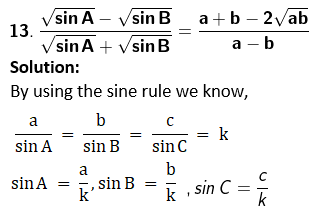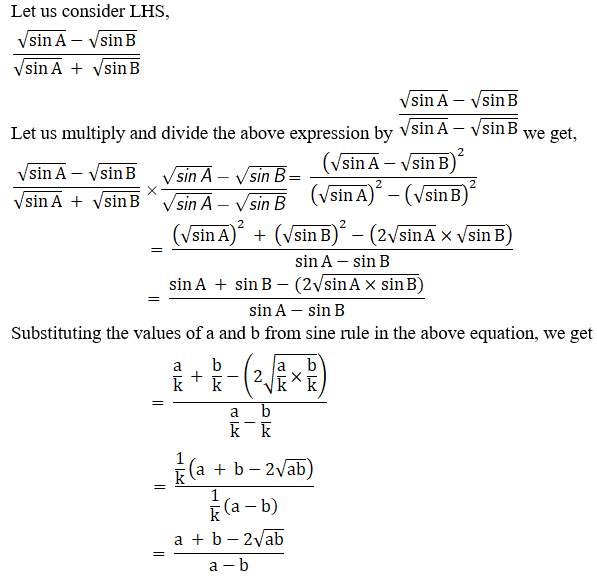= RHS

Hence proved.

14. a (sin B – sin C) + b (sin C – sin A) + c (sin A – sin B) = 0

Solution:

By using the sine rule we know,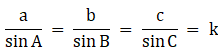a = k sin A, b = k sin B, c = k sin C

Let us consider LHS:

a (sin B – sin C) + b (sin C – sin A) + c (sin A – sin B)

Substituting the values of a, b, c from sine rule in above equation, we get

a (sin B – sin C) + b (sin C – sin A) + c (sin A – sin B) = k sin A (sin B – sin C) + k sin B (sin C – sin A) + k sin C (sin A – sin B)

= k sin A sin B – k sin A sin C + k sin B sin C – k sin B sin A + k sin C sin A – k sin C sin B

Upon simplification, we get

= 0

= RHS

Hence proved.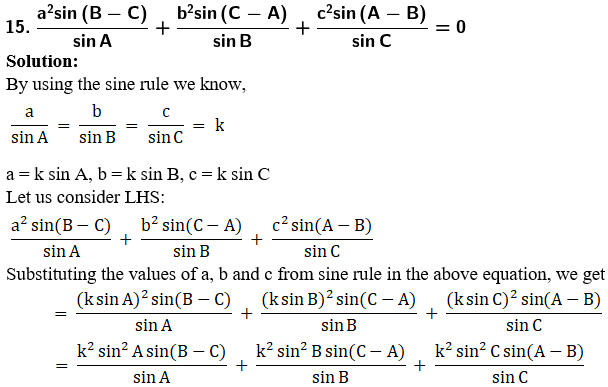Upon simplification we get,

= k2 [sin A sin (B – C) + sin B sin (C – A) + sin C sin (A – B)]

We know, sin (A – B) = sin A cos B – cos A sin B

Sin (B – C) = sin B cos C – cos B sin C

Sin (C – A) = sin C cos A – cos C sin A

So the above equation becomes,

= k2 [sin A (sin B cos C – cos B sin C) + sin B (sin C cos A – cos C sin A) + sin C (sin A cos B – cos A sin B)]

= k2 [sin A sin B cos C – sin A cos B sin C + sin B sin C cos A – sin B cos C sin A + sin C sin A cos B – sin C cos A sin B)]

Upon simplification we get,

= 0

= RHS

Hence proved.